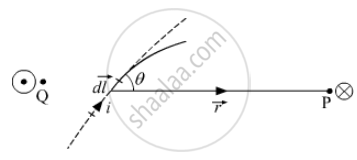Department of Pre-University Education, KarnatakaPUC Karnataka Science Class 12

# Express Biot – Savart Law in the Vector Form - Physics

Express  Biot – Savart law in the vector form.

#### Solution

Consider a straight infinnitely long wire carrying a steady current I. Point P is the perpenicular distance from the wire. Consider a small element dl of the wire at the point O on the wire. The line joining points O to P( OP = vector r ) makes an angle θ with the direction of the current element dl.dvecB = (μ_oI)/(4π) (vec(dl) xx vec r)/r^3

The expression for the total magnetic field B due to the wire can be obtained by intergrating the above expression as
vecB =∫(μ_oI)/(4π) (vec(dl)xxvecr)/r^3
It is the biot savart law which gives the magnetic field B generated by steady electric current I when the current can be approximated as running through an infinitely running wire.

Concept: Magnetic Field Due to a Current Element, Biot-Savart Law
Is there an error in this question or solution?